# Solution assignment 04 Logarithmic equations

### Assignment 4

Solve: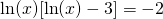### Solution

The domain is defined by the function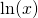and is equal to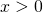(of course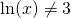, i.e.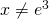, or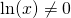, i.e.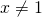).
We work out the equation: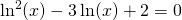We substitute into the equation: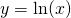and get: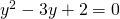This equation can be factorized: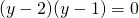and has the solutions: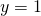or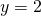Thus the corresponding solutions forare: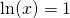and thus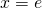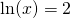and thus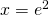Both solutions lie in the domain and are valid.